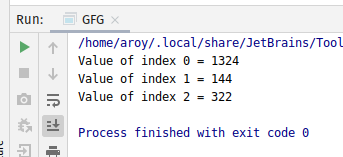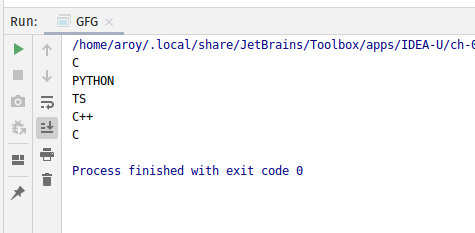# AtomicReferenceArray setRelease() method in Java with Examples

• Last Updated : 03 Jan, 2020

The setRelease() method of a AtomicReferenceArray class is used to set the value of the element at index i to newValue. Both index i and newValue are passed as parameters to the method. This method set the value with memory effects as specified by VarHandle.setRelease(java.lang.Object…). This method has memory ordering effects compatible with memory_order_release ordering.

Syntax:

```public final void setRelease(int i, E newValue)
```

Parameters: This method accepts:

• i which is an index of AtomicReferenceArray to perform the operation,
• newValue which is the new value to set.

Return value: This method returns nothing.

Below programs illustrate the setRelease() method:
Program 1:

 `// Java program to demonstrate``// setRelease() method`` ` `import` `java.util.concurrent.atomic.*;`` ` `public` `class` `GFG {`` ` `    ``public` `static` `void` `main(String[] args)``    ``{`` ` `        ``// create an atomic reference object.``        ``AtomicReferenceArray ref``            ``= ``new` `AtomicReferenceArray(``5``);`` ` `        ``// set some value and print``        ``ref.setRelease(``0``, ``1324``);``        ``ref.setRelease(``1``, ``144``);``        ``ref.setRelease(``2``, ``322``);`` ` `        ``System.out.println(``            ``"Value of index 0 = "``            ``+ ref.get(``0``));``        ``System.out.println(``            ``"Value of index 1 = "``            ``+ ref.get(``1``));``        ``System.out.println(``            ``"Value of index 2 = "``            ``+ ref.get(``2``));``    ``}``}`

Output:Program 2:

 `// Java program to demonstrate``// setRelease() method`` ` `import` `java.util.concurrent.atomic.*;`` ` `public` `class` `GFG {`` ` `    ``public` `static` `void` `main(String[] args)``    ``{`` ` `        ``// create an atomic reference object.c``        ``AtomicReferenceArray ref``            ``= ``new` `AtomicReferenceArray(``5``);`` ` `        ``// set some value``        ``ref.setRelease(``0``, ``"C"``);``        ``ref.setRelease(``1``, ``"PYTHON"``);``        ``ref.setRelease(``2``, ``"TS"``);``        ``ref.setRelease(``3``, ``"C++"``);``        ``ref.setRelease(``4``, ``"C"``);`` ` `        ``// print``        ``for` `(``int` `i = ``0``; i < ``5``; i++) {``            ``System.out.println(ref.get(i));``        ``}``    ``}``}`

Output:My Personal Notes arrow_drop_up